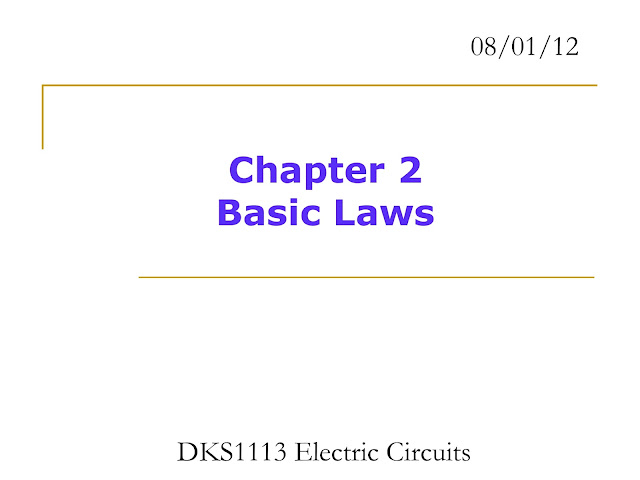## Monday, December 19, 2022

### BASICS ON ELECTRIC CIRCUIT - PDFIntroduction

Fundamental laws that govern electric circuits:

• Ohm’s Law.
• Kirchoff’s Law.

These laws form the foundation upon which electric circuit analysis is built.

Common techniques in circuit analysis and design:

• Combining resistors in series and parallel.
• Voltage and current divisions.
• Wye to delta and delta to wye transformations.
These techniques are restricted to resistive circuits.

Ohm’s Law

Ohm's law states that the current through a conductor is proportional to the voltage across the conductor. This is true for many materials (including metals) provided the temperature (and other physical factors) remain constant. The constant of proportionality, R,R is the resistance and the unit is the ohm, with symbol \Omega,Ω. The relationship can be written as: V, equals, I, R.

V=IR

where V,V is the voltage across the conductor and I,I is the current flowing through it. If a component is ohmic (it obeys Ohm's Law), then its resistance must be independent of current and voltage.